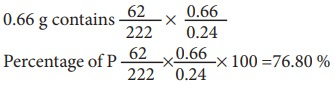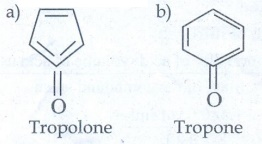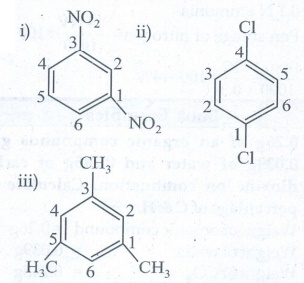Home | | Chemistry 11th std | Solved Example Problems: Fundamentals of Organic Chemistry

# Solved Example Problems: Fundamentals of Organic Chemistry

Solved Example Problems with solution or answers, Numerical Problems Questions with Answers, Solution : Chemistry: Fundamentals of Organic Chemistry

Fundamentals of Organic Chemistry

Numerical Problems Questions with Answers, Solution

## Worked out example 1

0.26g of an organic compound gave 0.039 g of water and 0.245 g of carbon dioxide on combustion. Calculate the percentage of C & H

Weight of organic compound = 0.26g

Weight of water = 0.039g

Weight of CO= 0.245g

### Percentage of hydrogen

18 g of water contain 2 g of hydrogen### Percentage of carbon

44 g of CO2 contain 12 g of C## Example -2

In an estimation of sulphur by carius method, 0.2175 g of the substance gave 0.5825 g of BaSO4 calculate the percentage composition of S in the compound.

Weight of organic compound 0.2175 g

Weight of BaSO4 0.5825 g

233 g of BaSO4 contains 32 g of S## Example 3:

0.284 g of an organic substance gave 0.287 g AgCl in a carius method for the estimation of halogen. Find the Percentage of Cl in the compound.

Weight of the organic substance = 0.284 g

Weight of AgCl is = 0.287 g

143.5 g of AgCl contains 35.5 g of chlorine## Example 4:

0.24 g of organic compound containing phosphorous gave 0.66 g of Mg2P2O7 by the usual analysis. Calculate the percentage of phosphorous in the compound

Weight of an organic compound       = 0.24 g

Weight of Mg2P2O= 0.66 g

222 g of Mg2P2O7 contains 62 g of P## Example 5:

0.1688 g when analyzed by the Dumas method yield 31.7 mL of moist nitrogen measured at 14º C and 758 mm mercury pressure. Determine the % of N in the substance (Aqueous tension at 14 º C =12 mm)### Example 6:

0.6 g of an organic compound was Kjeldalised and NH3 evolved was absorbed into 50 mL of semi-normal solution of H2SO4. The residual acid solution was diluted with distilled water and the volume made up to 150 mL. 20 mL of this diluted solution required 35 mL of N/20 NaOH solution for complete neutralization.

Calculate the % of N in the compound.

Weight of Organic compound = 0.6g

Volume of sulphuric acid taken = 50mL

Strength of sulphuric acid taken= 0.5 N

20 ml of diluted solution of unreacted sulphuric acid was neutralised by 35 mL of 0.05 N Sodium hydroxide

Strength of the diluted sulphuric acid = 35 × 0.05 / 20

= 0.0875 N

Volume of the sulphuric acid remaining after reaction with = V1 mL

Organic compound Strength of H2SO4 = 0.5N

Volume of the diluted H2SO4 = 150 mL

Strength of the diluted sulphuric acid = 0.0875 N

V1 = 150 ×0.087 / 0.5

= 26.25 mL

Volume of H2SO4 consumed by ammonia = 50 - 26.25 = 23.75 mL

23.75 mL of 0.5 N H2SO4 ≡ 23.75mL of 0.5N NH3

The amount of Nitrogen present in the 0.6 = 14g / [1000 mL × 1 N] × 23.75 × 0.5N = 0.166g

Percentage of Nitrogen = [0.166/0.6] × 100 = 27.66 %

Book back Exercise Problems

47. 0.30 g of a substance gives 0.88 g of carbon dioxide and 0.54 g of water calculate the percentage of carbon and hydrogen in it.

Weight of organic substance (w) = 0.30g

Weight of water (x) = 0.54g

Weight of CO2(y) = 0.88g

Percentage of carbon = (12/44) × (y/w) × 100

= (12/44) × (0.88/0.30) × 100

= 80%

Percentage of Hydrogen = (12/18) × (x/w) × 100

= (2/18) × (0.54/0.30) × 100

= 20%

48. The ammonia evolved form 0.20 g of an organic compound by kjeldahl method neutralised 15ml of N/20 sulphare acid solution. Calculate the percentage of Nitrogen.

Percentage of nitrogen = [ 1.4 × normality of acid × volume of acid used ] / [ Mass of the compound taken ]

= [ 1.4 × 0.05 × 15ml ] / 0.20

= 5.25%

49. 0.32 g of an organic compound, after heating with fuming nitric acid and barium nitrate crystals is a sealed tube game 0.466 g of barium sulphate. Determine the percentage of sulphur in the compound.

Weight of organic compound (w) = 0.32g

Weight of Barium sulphate (x) = 0.466g

Percentage of sulphur = (32/233) × (x/w) × 100

= (32/233) × (0.466/0.32) × 100

= 20.02%

50. 0.24g of an organic compound gave 0.287 g of silver chloride in the carius method. Calculate the percentage of chlorine in the compound.

Weight of organic compound (w)

= 0.24g

Weight of silver chloride (x) = 0.287g

Percentage of chlorine =

= (35.5 × 143.5) × (x/w) × 100

= (35.5/143.5) × (0.287/0.24) × 100

= 29.58%

51. In the estimation of nitrogen present in an organic compound by Dumas method 0.35 g yielded 20.7 mL of nitrogen at 150 C and 760 mm pressure. Calculate the percentage of nitrogen in the compound

Percentage of nitrogen = [ 28 / 22400 ] × [ Volume of N2 at STP / Weight of organic compound ] × 100

= [282/22400] × [20.7/0.35] × 100 = 7.39%

Evaluate Yourself

1. Give two example for each of the following type of organic compounds.

i) non - benzonoid aromatic

ii) aromatic heterocyclic

iii) alicyclicand

iv) aliphatic open chain

i) non - benzonoid aromaticii) Aromatic heterocycliciii) Alicyclic

a) Cyclo propane

b) Cyclobutane

iv) Aliphatic open chain

a) CH3 -CH2 - CH2 - CH3 = n - butane

b) CH3 CH2 - CH2 - CH2 - CH3 = n - pentane

2. Write structural formula for the following compounds.

i) Cyclohexa −1, 4 - diene

ii) Ethynyl cyclohexane

Ans :3. Write structural formula for the following compounds (March 19)

i) m - dinitrobenzene

ii) p - dichloro benzene

iii) 1, 3, 5 - Trimethyl benzene

Ans :4. Write all the possible isomers of molecular formula C4H10O and identify the isomerism found in them.

C4H10O Isomers5. 0.2346g of an organic compound containing C, H & O on combustion gives 0.2754g of H2O and 0.4488 g CO2 Calculate the % composition of C, H & O in the organic compound [C = 52.17, H = 13.04,O = 34.79]

[C = 52.17, H = 13.04, O = 34.79]

Weight of organic substance

(w) = 0.2346g

Weight of water (x) = 0.2754g

Weight of CO2 (y) = 0.4488g

Percentage of carbon = (12/44) × (y/w) × 100

= (12/44) × (0.4488/0.2346) × 100 = 52.17%

Percentage of hydrogen

= (2/18) × (x/w) × 100

= (2/18) × (0.2754/0.2346) × 100 = 13.04%

Percentage of oxygen = [100 - (52.17 + 13.04)]

= 100 – 65.21

= 34.79%

6. 0.16g of an organic compound was heated in a carius tube and H2SO4 acid formed was precipitated with BaCl2 . The mass of BaSO4 was 0.35g. Find the percentage of sulphur.

Weight of organic substance (w) = 0.16g.

Weight of Barium sulphate (x) = 0.35g

Percentage of Sulphur

= (32/233) × (x/w) × 100

= (32/233) × (0.35/0.16) × 100 = 30.04%

7. 0.185g of an organic compound when treated with Cone. HNO3 and silver nitrate gave 0.320g of silver bromide. Calculate the % of bromine in the compound. (Ag = 108, Br = 80)

Weight of organic substance (w) = 0.185g

Weight of silver bromide (x) = 0.320g

Percentage of bromine

= (80/188) × (x / w) × 100

= (80/188) × (0.32/0.185) × 100 = 73.6%

8. 0.40g of an iodo substituted organic compound gave 0.235 g of Agl by carius method. Calculate the percentage of iodine in the compound (Ag = 1081 = 127)

Weight of organic substance

(w) = 0.40g

Weight of silver iodide (x) = 0.235g

Percentage of iodine

= (127/235) × (x/w) × 100

= (127/235) × (0.235/0.40) × 100 = 31.75%

9. 0.33g of an organic compound containing phosphorous gave 0.397 g of Mg2P2O7 by the analysis. Calculate the percentage of P in the compound (MFW of Mg2P2Ois 222P = 31)

Weight of organic substance (w) = 0.33g

Weight of Mg2P2O7(x) = 0.397g

Percentage of phosphorus

= (62/222) × (x/w) × 100

= (62/222) × (0.397/0.33) × 100 = 33.59%

10. 0.3g of an organic compound on kjeldahl’s analysis gave enough ammonia to just neutralize 30 ml of 0.1 N H2SO4. Calculate the percentage of nitrogen is the compound.

Weight of organic substance(w) = 0.3g

Strength of H2SO4 used (N) = 0.1N

Volume of H2SO4 used (V) = 30 ml

30 ml of 0.1N H2SO4 = 30 ml of 0.1 N ammomia

Percentage of nitrogen = [ 14 NV/1000 ] × 100

[ 14 × 0.1 × 30 ] / [ 1000 × 0.3 ] × 100 = 14%

Tags : Chemistry , 11th Chemistry : UNIT 11 : Fundamentals of Organic Chemistry
Study Material, Lecturing Notes, Assignment, Reference, Wiki description explanation, brief detail
11th Chemistry : UNIT 11 : Fundamentals of Organic Chemistry : Solved Example Problems: Fundamentals of Organic Chemistry | Chemistry